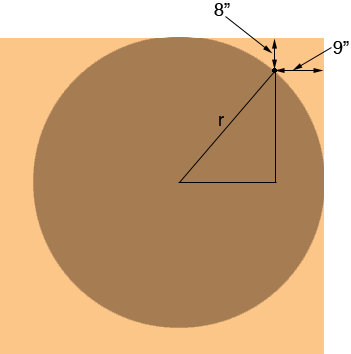SEARCH HOMEMath Central Quandaries & QueriesQuestion from Will, a student: A circular table is pushed into the corner of a square room so that one point is 8 inches from one wall and 9 inches from the other. What is the radius of the table?Hi Will,This looks like a Pythagoras Theorem problem. Do you see how to apply Pythagoras Theorem?

PennyMath Central is supported by the University of Regina and The Pacific Institute for the Mathematical Sciences.